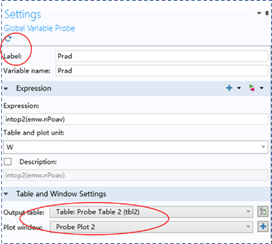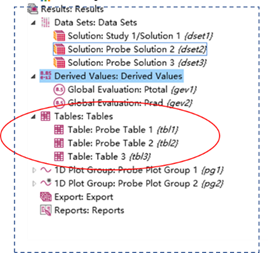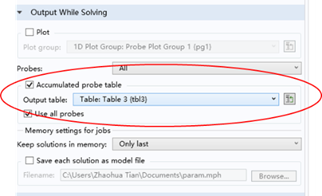# COMSOL

[TOC]

## Introduction

To summarize the problems we often encountered in using COMSOL. I will update it later.

## Problem 1 : No accumulated tables

• Problem description:

We need define some global variables (such as the total power and radiative power) and when we do parameter sweep in COMSOL, an accumulated table should be generated to store the global variable. However, in a certain case when we have many tables in COMSOL and even if we have chosen the “Accumulated Table” to be ”New”, the table is empty.

• Cause of problem:

This is due to the conflict of “table” and “plot window” for different global variable probe. Different probe may use the same “probe table” or “plot window” and will replace the content for each other.

• Solution to the problem:

1. Delete all tables, datasets, plot groups in COMSOL file.
2. Set the “output table” and “plot window” to be new and update (by typing the “⟳”) the global variable probe, respectively. Then all probe will have its own “probe table” and “plot window” and will not conflict with each other.
3. In the table menu, create new table and let the output table of the accumulated probe table to be the new created table.

A complete and correct settings for two probes should be as follows:## Problem 2: Can’t evaluate the electric field in the dipole’s position

• Problem description:

I want to update the electric field in the dipole’s position. There is no “point probe” to evaluate a certain point’s data. If we use the “domain point probe” or “boundary point probe”, the total power simulated from Poynting vector integral and electric field evaluation will be different. Actually, the result from “domain/boundary point probe” is wrong.

• Cause of the problem:

COMSOL will do interpolation in a domain or a boundary if we use “domain/boundary point probe” to evaluate a point’s data. In our case, the electric field near the dipole is very strong and the interpolation will let the electric field much smaller than the actual field.

• Solution to the problem:

The best way of evaluating a point’s data is by using COMSOL live link with MATLB. We use MATLAB to run and sweep COMSOL file and extract the data after each sweep process. To do this, we need learn:

1. How to run COMSOL file and do parameter sweep through MATLAB.
2. How to do point evaluation from MATLAB.
3. How to run COMSOL live link with MATLAB on server especially in the background.

We can learn above skills from the file “LiveLinkForMATLABUsersGuide.pdf” in COMSOL’s installation folder. I have written a note for 3 in the website

## Problem 3 : Please avoid the using of variable name “Lambda”

lambda has special meaning in COMSOL so you can’t use this name as your own variable.

• Problme description:

We may need use COMSOL livelink with MATLAB and the COMSOL file will be loaded into MATLAB through this livelink. Then we can’t modify this COMSOLfiel anymore only if we close the livelink server and restart it.

• Cause of the problem

This is becsause the mph file has been loaded into MATLAB through this sever and then is locked by this server. If we don’t close this file through this livelink we can’t use it any more.

• Solution to the problem

To solve this problem, we only need run the following command in MATLAB: